Courses

# Test: Linear Algebra - 9

## 20 Questions MCQ Test Topic-wise Tests & Solved Examples for IIT JAM Mathematics | Test: Linear Algebra - 9

Description
This mock test of Test: Linear Algebra - 9 for Mathematics helps you for every Mathematics entrance exam. This contains 20 Multiple Choice Questions for Mathematics Test: Linear Algebra - 9 (mcq) to study with solutions a complete question bank. The solved questions answers in this Test: Linear Algebra - 9 quiz give you a good mix of easy questions and tough questions. Mathematics students definitely take this Test: Linear Algebra - 9 exercise for a better result in the exam. You can find other Test: Linear Algebra - 9 extra questions, long questions & short questions for Mathematics on EduRev as well by searching above.
QUESTION: 1

### Let T be the linear operator on R3 defined by T(x1, x2, x3) = ( 2x1, x1 - x2, 5x1 + 4x2 + x3). Then T-1 is

Solution:

Let T be the linear operator on R3 defined by
T(x1 x2, x3) = (2x, x1 - x2, 5x1 + 4x2 + x3)
Let (x1, x2, x3) ∈ ker T.
Then we need to find T-1
Now, T(x1, x2, x3) - (0, 0, 0)
Using the definition of T, we get
(2x, xl - x2, 5x1 + 4x2 + x3) = (0, 0, 0)
Comparing the components of the coordinates, we get
2x1 = 0, x1 - x2 = 0, 5x1 + 4 x 2 + x 3 = 0
Solving for x1 x2 and x3, we get x1 = 0, x2 = 0, x3 = 0
Hence,ker T= {(0, 0, 0)}
and hence, T is one-one.
Since R3 is a 3-dimensional vector space and T : R3 —> R3 be a one-one linear transformation and hence, T is onto. Therefore, T-1 exist.
Let (a, b, c) be the image of (x1, x2, x3) under T-1.
Then T-1(x1,x2, x3) = (a, b, c) ImpliesT(a, b, c) = (x1,x2, x3)
Using the definition of T, we get
(2a a - b 5a + 4b + c) = (x1,x2, x3) Comparing the components, we get 2a = x1, a - b = x2, 5a l 4b I c = x3 Solving for a, b, c, we get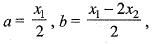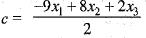Therefore, T-1(x1 x2, x3) =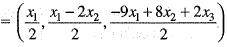QUESTION: 2

### The minimum polynomial m(λ) of M =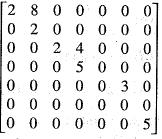Solution:

We are given that the matrix
M =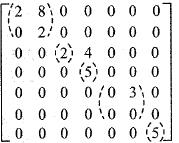We need to find the minimal polynomial of the given matrix. The characteristic polynomial of the given matrix is given by,Hence, the minimal polynomial of the given matrix M is,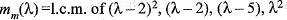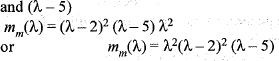QUESTION: 3

### The eigen values of a 3 x 3 real matrix A are 1,2 and - 3. Then

Solution:

We are given that the eigen values of a 3 x 3 real matrix A are 1,2 and -3 say λ1 λ2 and λ3.
The characteristic equation of the given matrix is,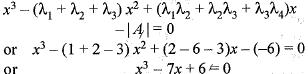By Cayley Hamilton’s theorem, we can write this equation in the matrix form as,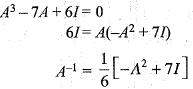QUESTION: 4

The minimal polynomial of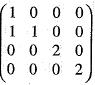is

Solution:

We need to find the minimal polynomial of the matrix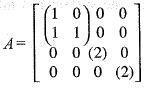The characteristic polynomial of the given matrix is,
chA(x) = (x - 1)2 (x - 2) (x - 2)
and the minimal polynomial of the given matrix
= l.c.m. of (x - 1)2, (x - 2) and (x-2)
= (x-1)2 (x-2)

QUESTION: 5

Let A be 3 x 3 matrix whose characteristic roots are 3 ,2 ,-1 .
If B = A2 - A, then |B| is

Solution:

We are given that A be a 3 x 3 matrix whose ' characteristic roots are 3, 2, -1 say λ1, λ2 and λ3 and B = A2 - A
λ1 = 3,
λ= (3)2 - 3 = 6
λ2 = 2,
λ2 = (2)2 - 2 = 2
λ3 = -1
λ3 = (-1)2 - (-1) = 2
The determinant of B is, | B | = product of its eigen values
= λ123'
= 6 x 2 x 2 = 24

QUESTION: 6

Let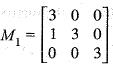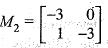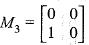Then, the minimal polynomial of the matrix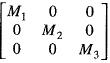is

Solution:

We are given that,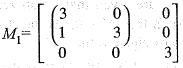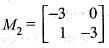and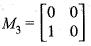We need to find the minimal polynomial of the matrix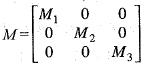The characteristic polynomial of the given matrix M is,
chm (x) =(x - 3)2 (x - 3) (x + 3)2 (x - 0)2
And the minimal polynomial of the given matrix M is
mx(x) = l.c.m. o f (x - 3)2,
(x - 3),(x + 3)2, (x - 0)2
or mm(x) = (x - 3)2 (x + 3)2x2
or mm (x) = x2(x - 3)2 (x + 3)2

QUESTION: 7

Let T : C—> Cn be a linear operator of rank n - 2. Then,

Solution:

We are given that a linear operator T : Cn—> Cn of rank n - 2.
Since T : Cn —> Cis a linear operator of rank n - 2.
Therefore, T is singular operator, Hence 0 must be an eigen value of T.

QUESTION: 8

Choose the correct matching from A, B, C and D for the transformation T1, Tand T3 (mappings from R2 to R3) as defined in Group 1 with the statements given in Group 2
Group 1
P.T1x( x, y) = (x , x, 0)
Q.T2(x,y) = (x, x +y ,y)
R.T3(x,y) = (x,x+1,y)
Group 2
1. Linear transformation of rank 2
2. Not a linear transformation
3. Linear transformation of rank 1

Solution:

Consider the linear transformation defined by
T1(x,y) = (x,x, 0)
Let (x1,x1) and (x2,x2​) be two vectors and α, β be scalars. Then
T1[α(x1 y1) + β(x2, y2)]
= T1[(αx1 + βx2, αy1 + βy2)]
= (αx1 + βx2, αx1 + βx2, 0)
= α(x1,x1, 0) + β(x2, x2, 0)
= αT1(x1,y1) + βT1(x2,y2)
or equivalently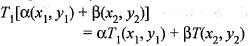Therefore, T1 is linear transformation.
Let (x,y) ∈ ker T1.
Then T(x,y) = (0, 0, 0)
Using the definition of linear transformation T1,we get
(x, x, 0) = (0, 0, 0)
Comparing the components of the co-ordinates, we get,
x = 0 and y is arbitrary
Therefore,ker T1{ = {(0, y ) : y ∈ R}
Hence,dim ker T1 = 1.
Using Rank nullity theorem Rank T1
= 2 - dim ker T
= 2 - 1 = 1.
Therefore, 'Tis a linear transformation of rank 1.
Next, consider the linear transformation T2 defined by
T2(x,y) = (x,x+y,y)
Let (x1,y1) and (x2,y2) be any two vectors and α, β are scalars.
Then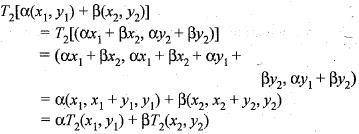Hence, T2 is a linear transformation.
Let (x, y) ∈ ker T.
Then T2(x,y) = (0, 0, 0)
Using the definition of linear transformation T2, we get
(.x, x+ y,y ) = (0, 0, 0)
Comparing the components of the coordinates, we get
x = 0, x + y = 0, y = 0
Solving for x and y, we get x = 0, y = 0
Hence,ker T2 = {(0,0)}.
Therefore,dim ker T2 = 0
Using rank nullity theorem,
Rank T2 = 2 - dim ker T2
= 2 - 0 - 2 .
Hence, T2 is a linear transformation of rank 2.
Next, consider the linear transformation T3 defined by
T3(x,y) = (x,x+1, y)
Then T3(0, 0) =(0, 1, 0)
but (0,1,0) is not a zero of codomain  space.
Hence, the image of (0, 0) under T3 is not a zero.
Therefore T3 is not a linear transformation.

QUESTION: 9

Let V, W and X be three finite dimensional vector spaces such that dim V = dim X, Suppose S : V --> W and T : W ---> X are two linear maps such ToS : V --> X is injective.Then,

Solution:

Let V, W and X be three finite dimensional vector spaces such that dim V = dim X. Suppose S : V --> W and T : W --> X are two linear maps such ToS : V --> X is injective.Then S is injective and T is surjective.

QUESTION: 10

​Let S and T be two linear operators on R3 defined by
S (x,y,z) = (x,x + y , x - y - z )
T(x , y , z) = (x + 2z ,y - z ,x + y + z)

Solution:

Let S and T be two linear operators on R3 defined by
S(x, y, z) = (x, x + y, x - y - z)
and T(x, y, z) = (x + 2z, y - z, x + y + z).
Let (x, y, z) ∈ ker S.
Then S(x,y,z)= (0,0,0)
Using the definition of linear transformation S, we get
(x , x + y , x - y - z ) = (0, 0, 0) Comparing the components of the coordinates, we get
x = 0 , x+ y = 0, x - y - z = 0
Solving for x, y and z, we get
x = 0, y = 0, z = 0
Hence,ker S = {(0, 0, 0)}
Therefore, S is one-one.
Since S is a one-one linear operator on R3. Therefore, S is onto and hence invertible that is non-singular.
Next, Let (x, y, z) ∈ ker T. Then
T(x,y,z)=(0,0,0)
Using the definition of linear transformation T, we get
(x + 2z ,y - z , x + y + z) = (0, 0, 0) Comparing the components of the coordinates, we get
x + 2 z = 0 , y - z = 0, x + y + z = 0 Solving for x, y and z, we get x = -2z, y = z Therefore, ker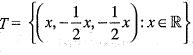Hence, dim ker T= 1. Therefore, T is not one-one and hence T is not invertible that is T is singular.

QUESTION: 11

Let A be the matrix of quadratic form (x1- x2 + 2x3)2.
Then, trace of A is

Solution:

We are given that A be a matrix of quardratic form (x1-x2 + 2x3)2
or x12 + x22 + 4x32 - 2x1x2 - 4x2x3 + 4x3x1
trace (A) = sum of coefficient of
x12, x22 and x32.
= 1 + 1 + 4
= 6

QUESTION: 12

The eigen values of a 3 x 3 real matrix P are 1, -2, 3. Then,

Solution:

We are given that the eigen values of a 3 x 3 real matrix P are 1, - 2 ,3 say λ1, λ2, λ3.
The characteristic equation of the given matrix P is,
x2 - (trace P) x2 + (λ1•λ2 + λ2•λ3 + λ3•λ1)x - |P| = 0
or x3 - (1 - 2 + 3)x2 + (-2 - 6 + 3) x -(-6) = 0
or x3 — 2x2 — 5x + 6 = 0
By Cayley Hamilton theorem, we can write this equation in the matrix form.
p3 - 2P2 - 5p + 6I = 0
or 6I = 5P + 2P2 - P3
or 6I = P(5I + 2P - P2)
or P-1 = 1/6(5I + 2P - P2)

QUESTION: 13

The system of equations
x + y + z = 0
3x + 6y + z = 0
αx + 2y + z = 0
has infinitely many solutions, then a is equal to

Solution:

We are given that the system of equations,
x + y+z= 0
3x + 6y + z = 0
αx + 2y + z = 0
has infinitely many solutions. We need to find the value of a. The given system of equation may be written in matrix form as,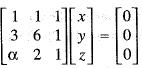Reduce this system of equation to echelon form using the operations
‘R2 --> R2 - R1' and R3 ---> R3 - R1, these operations yields -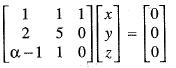Also applying , R3 —> 5R3 - R2 we get -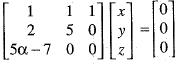Since, this system of equations has infinitely man solution. It is possible when 5α - 7 = 0
or α = 7/5

QUESTION: 14

Consider the system of linear equation
x + y + z = 3, x - y - z = 4, x - 5 y + kz = 6
Then, the value of k for which this system has an infinite number of solution is

Solution:

We are given that the system of equations,
x + y +z = 3
x - y - z = 4
x - 5y + kz = 6
has infinitely many solution and we need to find the value of k. The given system of equation may be written in the matrix form as,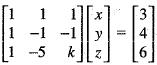Reduce this system of equations to echelon form using the operations "R2 —> R2 - R1", "R3 --> R3 - R1" these operations yield -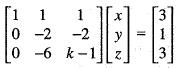and also applying "R3 -> R3 - 3R2" we get.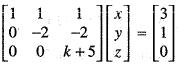Since, the system has infinite solution it is possible when,
k + 5 = 0 or k = - 5

QUESTION: 15

Real matrices [A]3x1,[B]3x3, [C]3 x 5 [D]5 x 3, [E]5 x 5 and [F]5 x 1, are given. Matrices [B] and [E] are symmetric. Following statements are made with respect to these matrices:
(i) Matrix product [F]T [C]T [B] [C] [F] is a scalar.
(ii) Matrix product [D]T [F] [D] is always symmetric. With reference to above statements, which of the following applies?

Solution:
QUESTION: 16

For any two matrices A and B, which is true?

Solution:
QUESTION: 17

Matrix A =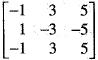is

Solution:
QUESTION: 18

Matrix A =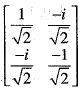is

Solution:
QUESTION: 19

Match List I with List II and select the correct answer using the codes given below in the lists.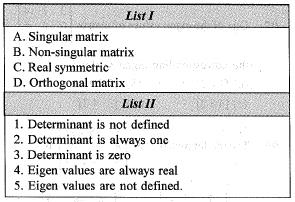Codes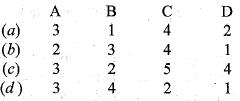Solution:
QUESTION: 20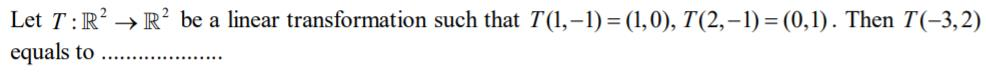Solution: